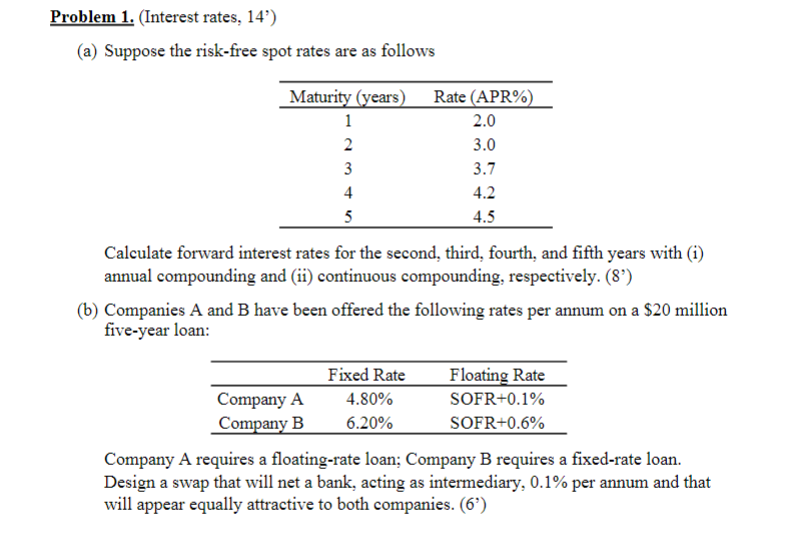Home / Expert Answers / Finance / a-suppose-the-risk-free-spot-rates-are-as-follows-calculate-forward-interest-rates-for-the-second-pa392

# (Solved): (a) Suppose the risk-free spot rates are as follows Calculate forward interest rates for the second ...(a) Suppose the risk-free spot rates are as follows Calculate forward interest rates for the second, third, fourth, and fifth years with (i) annual compounding and (ii) continuous compounding, respectively. (8') (b) Companies and have been offered the following rates per annum on a million five-year loan: Company A requires a floating-rate loan; Company B requires a fixed-rate loan. Design a swap that will net a bank, acting as intermediary, per annum and that will appear equally attractive to both companies. (6')

We have an Answer from Expert